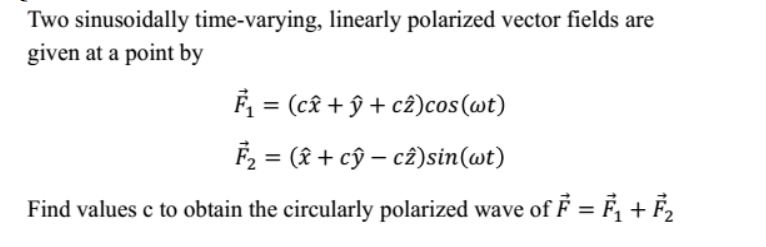Home / Expert Answers / Electrical Engineering / two-sinusoidally-time-varying-linearly-polarized-vector-fields-are-given-at-a-point-by-begin-pa897

# (Solved): Two sinusoidally time-varying, linearly polarized vector fields are given at a point by $\begin{ ...Two sinusoidally time-varying, linearly polarized vector fields are given at a point by \[ \begin{array}{l} \vec{F}_{1}=(c \hat{x}+\hat{y}+c \hat{z}) \cos (\omega t) \\ \vec{F}_{2}=(\hat{x}+c \hat{y}-c \hat{z}) \sin (\omega t) \end{array}$ Find values $$\mathrm{c}$$ to obtain the circularly polarized wave of $$\vec{F}=\vec{F}_{1}+\vec{F}_{2}$$

We have an Answer from Expert